# One-Step Division Equations - MathHelp.com - Math Help

##By yourteachermathhelp

1000+ online math lessons featuring a personal math teacher inside every lesson! In this lesson students learn to solve one-step division equations. For example to solve x/4 = 2 multiply both sides of the equation by 4 to get x = 8. Next check the solution by substituting an 8 back into the original equation to get (8)/2 = 4 which is a true statement so the solution checks.# One-step equations with multiplication and division

##By Khan Academy

Remember that what you do to one side, you have to do to the other. Will you multiply or divide both sides to dump the fraction, x/a? Let's do it together.# One-step equations with multiplication and division

##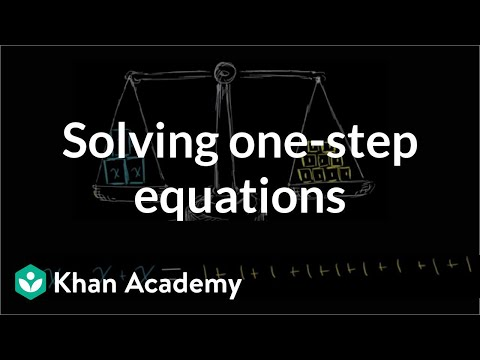By Khan Academy

This equation can be simplified through a single step to solve for the variable. Can you help?# One-step equations with multiplication and division

##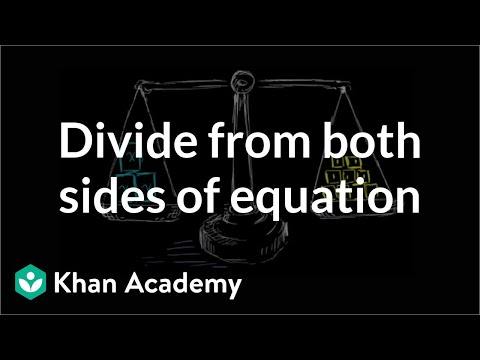By Khan Academy

Let's get a conceptual understanding of why one needs to divide both sides of an equation to solve for a variable.# One-step equations with multiplication and division

##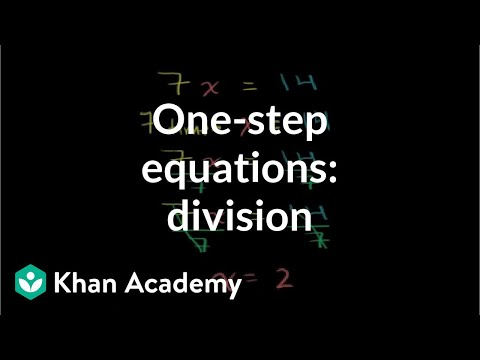By Khan Academy

Let's ease into this, shall we? Here's an introduction to basic algebraic equations of the form ax=b. Remember that you can check to see if you have the right answer by substituting it for the variable!# One-step equations with multiplication and division

##By Khan Academy

This equation can be simplified through a single step to solve for the variable. Can you help?# One-step equations with multiplication and division

##By Khan Academy

Let's ease into this, shall we? Here's an introduction to basic algebraic equations of the form ax=b. Remember that you can check to see if you have the right answer by substituting it for the variable!# One-step equations with multiplication and division

##By Khan Academy

Let's get a conceptual understanding of why one needs to divide both sides of an equation to solve for a variable.# One-step equations with multiplication and division

##By Khan Academy

Remember that what you do to one side, you have to do to the other. Will you multiply or divide both sides to dump the fraction, x/a? Let's do it together.# One-step multiplication and division equations with fractions and decimals

##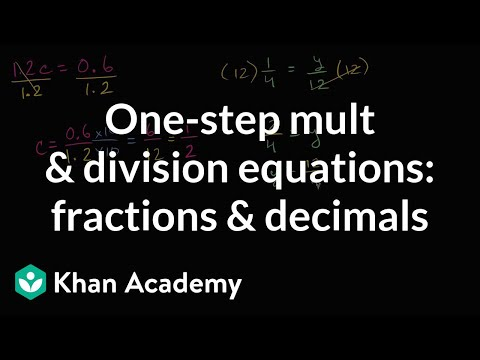By Khan Academy

Learn how to solve equations in one step by multiplying or dividing a number from both sides.ï¿½ï¿½ï¿½ï¿½ï¿½ï¿½ï¿½ï¿½ï¿½ï¿½ï¿½ï¿½These problems involve decimals and fractions.# Unknowns with multiplication and division | Multiplication and division

##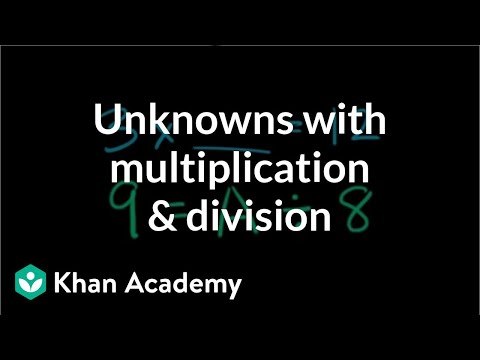By Khan Academy

Find the missing number in multiplication and division equations.# Finding the Missing Factor

##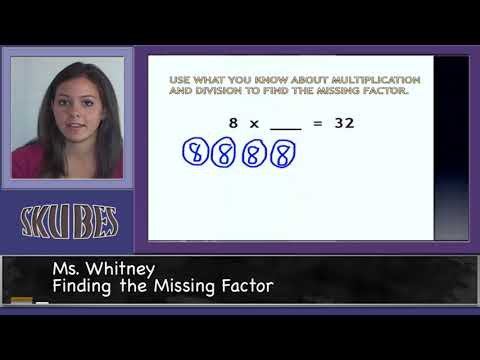By Skubes ed

Determine the unknown whole number in a multiplication or division equation relating three whole numbers. For example, determine the unknown number that makes the equation true in each of the equations 8 x ? = 48, 5 = _ ÷ 3, 6 x 6 = ?# [3.OA.4-2.0] Unknowns in Mult/Div - Common Core Standard

##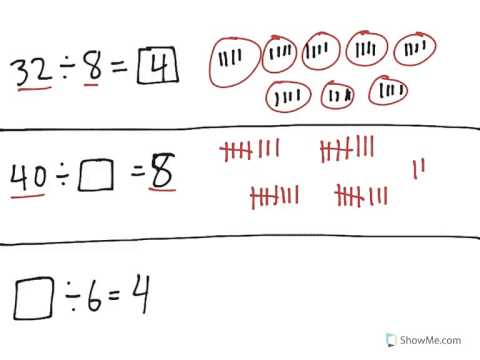By Freckle education

Determine the unknown whole number in a division equation relating three whole numbers. For example, determine the unknown number that makes the equation true in each of the equations 8 × ? = 48, 5 = _ ÷ 3, 6 × 6 = ?# One-step multiplication and division equations with fractions and decimals

##By Khan Academy

Let's ease into this, shall we? Here's an introduction to basic algebraic equations of the form ax=b. Remember that you can check to see if you have the right answer by substituting it for the variable!# One-step multiplication and division equations with fractions and decimals

##By Khan Academy

Remember that what you do to one side, you have to do to the other. Will you multiply or divide both sides to dump the fraction, x/a? Let's do it together.# Division - Sharing Equally: Grade 3

##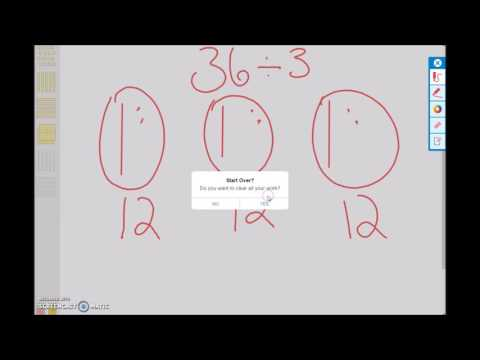By university of Houston mathematics education

Use multiplication and division within 100 to solve word problems in situations involving equal groups, arrays, and measurement quantities, e.g., by using drawings and equations with a symbol for the unknown number to represent the problem.# Division - Sharing Equally: Grade 3

##By university of Houston mathematics education

Use multiplication and division within 100 to solve word problems in situations involving equal groups, arrays, and measurement quantities, e.g., by using drawings and equations with a symbol for the unknown number to represent the problem.# Division Problem Solving

##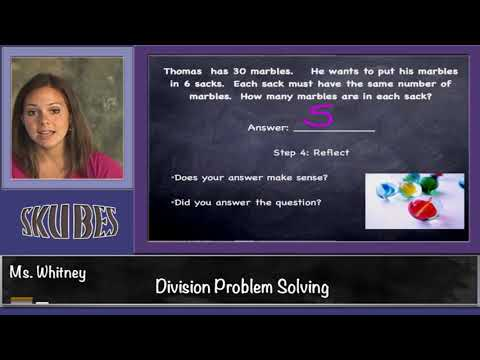By Skubes ed

Use multiplication and division within 100 to solve word problems in situations involving equal groups, arrays, and measurement quantities, e.g., by using drawings and equations with a symbol for the unknown number to represent the problem.1# Reasoning with Equations and Inequalities

##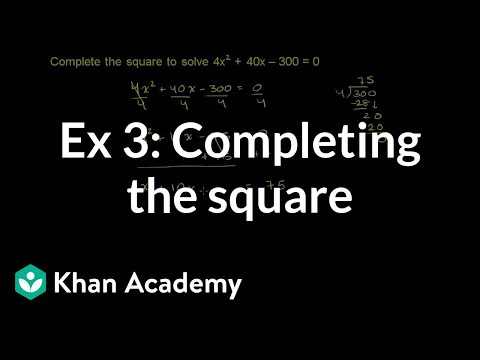By Khan Academy

Sal solves the equation 4x^2+40x-300=0 by completing the square.265# Composing equations and inequalities

##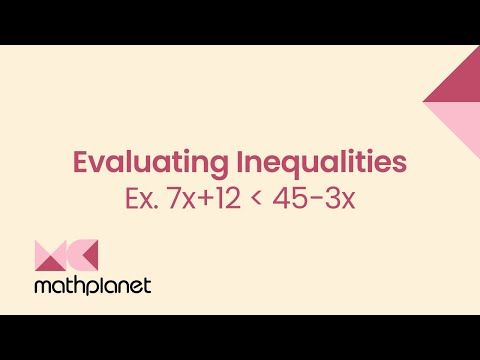By MathPlanetVideos

Is 3 a solution to 7x+12<45−3x